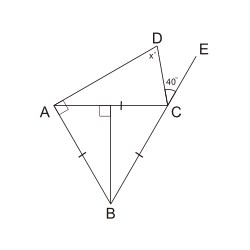We cover every section of the GMAT with in-depth lessons, 5000+ practice questions and realistic practice tests.

## Up to 90+ points GMAT score improvement guarantee

### The best guarantee you’ll find

Our Premium and Ultimate plans guarantee up to 90+ points score increase or your money back.

## Master each section of the test

### Comprehensive GMAT prep

We cover every section of the GMAT with in-depth lessons, 5000+ practice questions and realistic practice tests.

## Schedule-free studying

### Learn on the go

Study whenever and wherever you want with our iOS and Android mobile apps.

# Triangles: EquilateralIf $$\triangle ABC$$ is an equilateral triangle, what is the value of $$x$$?
Incorrect. [[snippet]] Carefully check your calculations.
Correct. [[snippet]] Since $$AB = AC = BC$$, >$$\angle ABC = \angle BCA = \angle CAB = \frac{180^\circ}{3} = 60^\circ$$ Based on this, >$$\angle ACD = 180^\circ - 60^\circ - 40^\circ = 80^\circ$$. Given that $$\angle CAB = 60^\circ$$, >$$\angle CAD = 90^\circ - 60^\circ = 30^\circ$$. So, >$$x = 180^\circ - 80^\circ - 30^\circ = 70^\circ$$.
Incorrect. [[snippet]] Carefully check your work.
Incorrect. [[snippet]] You might have gotten this answer if you assumed that $$\angle ACD$$ is a right angle.
Incorrect. [[snippet]] You may have gotten this answer if you tried to use the properties of parallel lines. Lines $$AD$$ and $$CE$$ are _not_ parallel.
20°
40°
60°
70°
80°